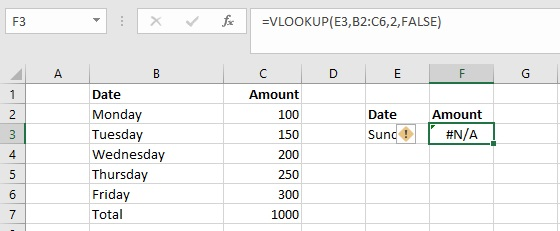# Excel Formula Errors and How to Fix Them

## Excel Errors with solution

This post will be covering frequently occurring formula errors in excel and solution for each of these errors.

## 1. ######

It is not an error. this means that the result length is more than the width of the column.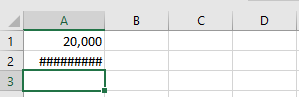Solution: Extend the width of the column.

### 2.# Div/0! error

This error happens when a number is divided either by zero (0) or an empty cell.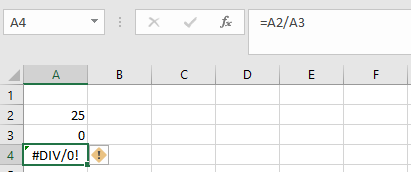A2/A3 =25/0 // Error #DIV/0!

Solution: Change the divider to a value that is not equal to 0 or empty cell.

### 3.#Name? error

This error happens if in case the formula is not typed correctly.For Example =su(B2:B3) will result in the #Name? error.

Solution: Correct the error by entering =SUM (B2:B3). (Here, add only B2+B3)

### 4.#Value! error

This error will be displayed if the formula includes cells that contain different data types.Solution: Replace NA with a numeric value and the error will be corrected. Or Change the value of cell C2 to a number.

MONTH function can’t extract a month value from “apple”, since “apple” is not a date.

date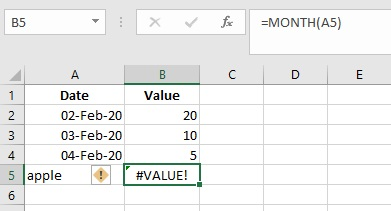Solution: Replace apple with a date(05-Feb-2020)

### 5.#REF! error

If in case of missing reference, this error will happen. Or Deleting cells that were referred by other formulas will cause this error.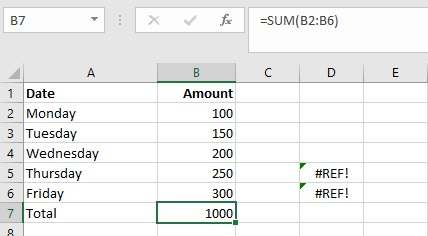Above screen, the formula in B7 was copied to D5 or D6. At this new location, since the range B2:B6 is relative, it becomes invalid and the formula returns #REF!: don’t copy to the another cell.

Solution: Refer the cells to the correct ranges and the error will be corrected.

### 6.#NULL! error

The #NULL! error is usually the result of a typo where a space character is used instead of a comma (,) or colon (:) between two cell references.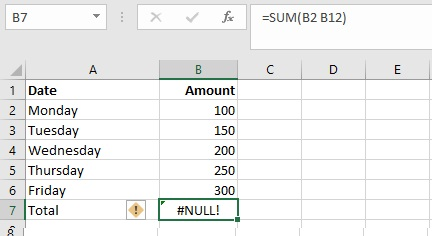=SUM (B2 B12) // returns #NULL!

Solution: change to SUM (B2:B6) not B12 and also colon is added, the error is fixed

=SUM (B2:B6) // after fix the error

### 7.#NUM! error

This error happens when Excel encounters an invalid number.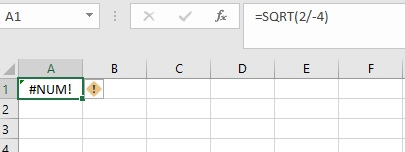Here, SQRT (2/-4) not valid // #NUM! (it is not possible to compute the square root of a negative division number.

Solution: enter only positive numbers (For SQRT function)

### 8. #N/A

This error is produced when a value is not available to your formula. The functions mostly commonly affected by the #N/A error are classic lookup functions, including VLOOKUP, HLOOKUP, LOOKUP, and MATCH.

Below ,the formula in F3 returns #N/A because “Sunday” is not in the lookup tablethe formula in F3 returns #N/A because “Sunday” is not in the lookup table

Solution: change to mon or tue,etc.